# Investigation of Thermo-Hydraulics Flow and Augmentation of Heat Transfer in the Circular Pipe by Combined Using Corrugated Tube with Dimples and Fitted with Varying Tape Insert Configurations

Investigation of Thermo-Hydraulics Flow and Augmentation of Heat Transfer in the Circular Pipe by Combined Using Corrugated Tube with Dimples and Fitted with Varying Tape Insert Configurations
Department of Mechanical Engineering, Faculty of Engineering, Mustansiriyah University, Baghdad 10011, Iraq

Washington State University, School of Mechanical and Materials Engineering, WSU Tri-Cities 99304, USA

Corresponding Author Email:
ahmedram@uomustansiriyah.edu.iq
Page:
365-374
|
DOI:
https://doi.org/10.18280/ijht.390205
26 November 2020
|
Accepted:
5 March 2021
|
Published:
30 April 2021
| Citation

OPEN ACCESS

Abstract:

In various industrial applications, the high performance of heat exchanger demand is increasing. Subsequently, the energy resources depletion, for instance, in power plant, air-conditioning system and food processing systems. The important field for saving energy was through improving thermal performance, which can provide high performance heat exchanger. Present enhancing approaches can be classified by three changed types, which are passive technique, active technique and compound technique. Dimple, twisted tape and corrugated pipe are the passive heat improvement technique which includes more surface extensions. Hence, this research work concentrates on verifying the computational calculations of flow in the heat exchanger pipe with different surface extensions in the pipe. It is carried out for turbulent flow with a range of Reynolds number from 1000 to 15000 using CFD methods. The numerical outcomes illustrate that change twisted tape configurations have more effect on flow and heat performance. Experimental and numerical results agreement can confirm the simulation technique reliability, which adopts in this investigation. The deviation errors are observed by less than 6% compared with the normal pipe. Pressure drop increases due to the rise of twisted tape dimensions (width and thickness), leading to more mixing of fluid, secondary flow, and swirl flow inside the pipe. As the tape geometrical parameters increase, the f value also increases due to more variance in velocities flow between liquid layers, which are adjacent to tape surfaces a pipe wall, and pipe core flow layers, become higher. Correspondingly, compared to the normal pipe, twisted configurations can rise f about 5.4 to 33.5%. The better thermal evaluation factor is at a twisted tape of 1x1 mm at Re number of about 1000. The range value of the thermal evaluation factor is more than 1.67.

Keywords:

thermo-hydraulics flow, augmentation of heat transfer, corrugated tube, dimples, tape insert configurations

1. Introduction

Different types of heat exchangers have been widely utilized in numerous industrial applications [1, 2]. In order to raise economic advantage and decrease energy consumption, heat transfer improvement techniques are becoming more generally used in various fields [3, 4]. The enhancement of heat transfer techniques was categorized into different types, such as passive, active, and compound classified. Generally, the passive techniques become used in many geometry types surfaces to improve thermal performance without used any additional power supply [5, 6]. So, such approaches have been extensively applied in industry. To enhance heat performance in the system, various passive devices were employed consisting of twisted taps, ribs, fins, insert the device, dimples, wire coils, corrugated surfaces, etc. [7, 8]. These devices can highly increase the flow separation, flow mixing, disruption in the boundary layer and turbulence and hence raise the performance of heat transfer [9, 10]. Additional heat transfer rate improvements in heat exchanger pipes have high importance in many industrial applications. Numerous various passive methods exist to raise the rate of heat transfer frequently for straight tubes. In the model of passive methods, heat transfer improvement through chaotic flow mixing in corrugated tubes has high importance is studied. In the last decades, the use of combined corrugated tubes, dimples and tape has been made to develop heat transfer enhancement to improve the heat exchanger's performance and reduce the size and cost. Several researchers numerically and experimental investigate the heat transfer characteristics by combined using the corrugated tube with dimples and fitted with varying inserted tape [11-14]. In the literature, the applications of tubular heat exchangers with inserted twisted-tape, as a passive technique for heat transfer enhancement, have been widely studied. Chen et al.  experimentally investigate the effect of different dimpled copper tube geometries in turbulent flow with Reynolds numbers in the range of 7500 to 52000, and water is the working fluid to heat transfer enhancement. They concluded that with a constant Reynolds number, the heat transfer enhancement was up to 137%. At constant pumping power, the heat transfer enhancement was up to 84%. They found that the dimpled tube improvement in mechanical strength and resistance to thermal expansion and the enhancement can reduce the heat exchanger's size and weight by a factor of 2 without affecting the system. Kukulka and Smith  conducted experiments on enhanced pipes to evaluate the relationship between heat transfer enhancement and tubes' surface geometry with Reynolds Number up to 2300. They concluded that heat transfer enhancement by 500% with compression to smooth pipes, reducing the heat exchanger operating cost and size. Experimental data have been reported by Liao and Xin  to study the heat transfer characteristics and friction inside 3d internal extended surfaces with inserted twisted tape, or by replacing continuous twisted tape with segmented twisted tape, using different working fluids such as water and ethylene glycol, using various ranges of Prandtl and Reynolds numbers, they concluded that the average Stanton number increased by a factor of 6.5 with compression to the smooth pipes this will lead to heat transfer and friction factor enhancement. Patil  experimentally studied the friction and heat transfer characteristics in a circular tube. The experiments were conducted with different width twisted tape inserted and with a range of Re No. up to 500 (laminar swirl flow). He found that using varying width twisted tapes, up to 60% less friction factor than twisted tapes with full length, was obtained also observed that the Nusselt number reduced by up to 25% at uniform wall temperature 50% reduction in tape-width. Using a twisted tape insert in the corrugated tube has been found in many preliminary experimental results that increased heat transfer performance [19-21]. Extensive research has been done by Saha et al.  to study the pressure drop and heat transfer characteristic of a circular tube fitted with tape connected with a rod. He concluded that the pressure drop is 40% smaller for the tube fitted with the segmented twisted tape than the tube fitted with a continuous twisted tape. He found that the twisted tape insert generates a considerable increase in heat transfer. Dimpled tube with twisted tape was employed by Thianpong et al.  for heat transfer enhancing, they investigated the thermal-hydraulic performance of combinations number of twisted tapes and dimpled tubes, based on their results, they presented the empirical correlations for prediction of Reynolds number range of (12000 – 44000) to find the Nusselt number and friction factor, The concluded that the heat transfer rate and friction factor in the dimpled tube with twisted tape affected by the pitch and twist ratios, the heat transfer rate of the enhanced tube with twisted tape insert was increased by a factor of 3.03 and friction factor by a factor of 6.31 compared with smooth pipes. Experimentally Investigation by Promvonge and Eiamsa-ard  of the heat transfer performance of the conic ring and twisted-tape, they found that the average heat transfer performances increased by 367% compared to a plain tube, they concluded that this technique had better heat transfer performance than using conical-ring. Also, they found that the heat transfer rate increases considerably for using both enhancement devices, up to 50% higher than using a single enhancement device in similar operating conditions. In addition, the friction factor from using both devices also increased considerably. An investigation study of the heat transfer, friction factor, and enhancement efficiency characteristics have been carried out by Eiamsa-ard et al.  for the round pipe with short-length twisted tape swirl. They observed that the short length tape has advantages in the reduction of the friction and saving materials; also, lower thermal performance for the short-length tape insert compared to full-length tape has been observed during the experiment. Besides the experimental investigation, a numerical investigation is also carried out by Zhang et al.  to investigate the heat transfer performance of flow in the tube with helical screw-tape with different widths (7.5, 12, 15 and 20 mm). The simulation results show that the thermal performance factor varied from 1.58 to 2.35. They reported that the average overall heat transfer coefficients increase by about 351% by inserting helical screw-tape in a circular tube, and the friction factor is increased by 33-1020%.

Numerically Investigation by Ray and Date  to predict the heat transfer characteristics in twisted tape inserted in square ducts, the twisted tape contacts with and away from the wall laminar and turbulent flow. The heat transfer characteristics were numerically predicted under heat flux conditions, correlations for friction factor and Nusselt number with Reynolds number range of 40 to 1100 for laminar and Reynolds number range of 4000 to 60000 for turbulent flow were proposed. A three-dimensional finite element model was developed by Al-Obaidi and Alhamid  using ANSYS to study the friction factor characteristics and heat transfer of laminar flow in a circular tube fitted with twisted tape. They found that the thermo-hydraulic performance is low for the tubes with short-width twisted tapes, and the flow resistance can be decreased in the tube with narrow-width and center-cleared twisted tapes. This technique is promising for heat transfer enhancement for laminar flow. Their results show that the heat transfer enhancement increased by 20% compared to the tube with conventional twisted tape.

A numerical investigation by Bellos and Tzivanidis  examined the use of internal fins, twisted tape inserts, and perforated plate inserts in evacuated and non-evacuated tube collectors with a flow rate of the working fluid are 100 L/min. The numerical results showed that the thermal efficiency enhancement of internally finned absorber for non-evacuated, evacuated tube collector, the twisted tape for non-evacuated and for evacuated tube collector 2.1%, 1.6%, 1.8%, 1.5% respectively.

Also, the reported that the pressure losses would increase when the absorber contains internal fins. In contrast, the use of perforated tape inserts showed less thermal efficiency enhancement. A Computational Fluid Dynamic (CFD) for the heat transfer in a tube fitted with alternative axis twisted tape with different ratio and locations in laminar flow under a constant heat flux was investigated by Salman et al. . The tube was equipped with twisted tape inserts with different alternative angles (30°, 60°, and 90°) for laminar flow at uniform heat flux. The numerical results showed that the twist ratio of 2.93 with an angle of 90ᵒ offered a maximum heat transfer enhancement. So, the improvement in heat performance fields have more advantage in industrial applications, particularly in forced heat transfer convective arena.

The main important reasons for using heat improved approaches are attempted to save the costs and for applied purposes. One of the general enhancements of heat transfer is the dimple, corrugated surfaces, ribs, grooves, and twisted tape. From the above literature review and experimental investigations, the modified corrugated tube with dimples and fitted with varying tape inserts offered better thermal performance than typically twisted tapes.

2. Description of Geometrical Physical Model Configurations

Passive methods using different techniques such as dimple, corrugation and twisted tap configurations are progressively becoming more effective approaches for obtaining higher efficiency in pipes heat exchangers and decreasing costs. The pipe length of 1920 mm, pipe diameter of 11.08 mm with 2 mm thickness, is simulated using different types of configurations based on the CFD technique. In this analysis, twisted tape configurations are changing with dimple and corrugation configurations kept constant. The main twisted tape corrugation parameters for width and thickness including (1 mm x 1 mm, 1.5 mm x 1.5 mm, 2 mm x 2 mm, 2 mm x 3 mm, 2 mm x 4 mm, 2 mm x 5 mm and 2 mm x 6 mm) and dimple diameter of 0.5 mm as well as corrugated ring diameter of 1 mm as presented in Figure 1. The numerical modelling flow domain of the pipe is described in this figure. The inlet and outlet pipe are applied to develop flow in order to decrease backflow fully. The water fluid properties are specific heat of 4178 J/kg.K, the thermal conductivity of 0.63 W/m.K, density of 992.32 kg/m3 and dynamic viscosity of 6.71x10-4 Ns/m3, respectively.

## 1.png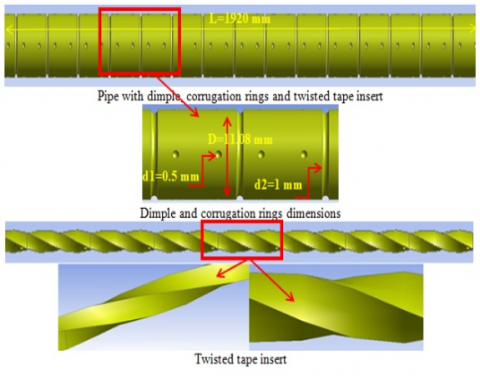## 1.2.png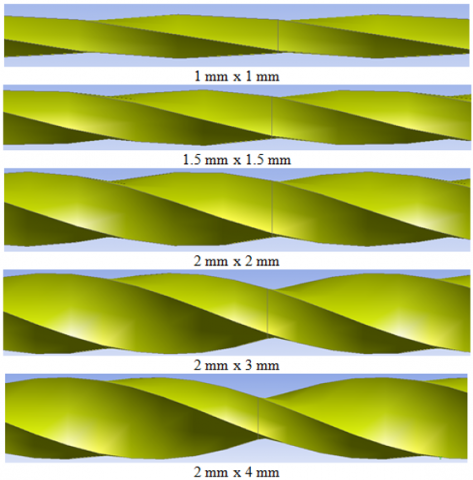## 1.3.pngFigure 1. Schematic diagram for the pipe with dimple, corrugation rings and twisted tape configurations

3. Flow Mesh Domain Parameters

The meshes types of refined unstructured tetrahedral with prismatic at pipe wall elements are created in this numerical flow model. Process of flow meshing applying three various mesh element sizes to investigate the utmost accurate and reliable outcomes and tetrahedral nodes types are chosen for further analysis. It is followed through the criteria of meshing, for instance, inflation layers and mesh quality. The computation calculations accuracy and stability are mostly dependent on meshing quality, which includes an important aspect ratio and skewness setting for mesh domain. Latter mesh qualities play a significant part to make ensure the mesh elements are good as well as in order to evade simulation calculation error. For that case, aspect ratio and skewness are 16 and 0.8, respectively. Checking the grid characteristics is very essential, notwithstanding the meshing kinds used in the flow domain. The description of all flow domain for both smooth and pipe with a dimple, corrugated surfaces and twisted tape is depicted in Figure 2. In this research, various meshes generations are carried out comprising of 1.5 million, 2.5 million, and 3.5 million elements as exhibited in the below Table 1. The outcomes of Nu number are used to compare between these three cases the changes in the value of Nu is less than 1.97% for 3.5 million elements.

Table 1. Investigation of mesh independency

 No. of elements Nu Difference in Nu number (million) (-) (%) 1.5 14.5 - 2.5 14.9 2.68 3.5 15.2 1.97

## 2.png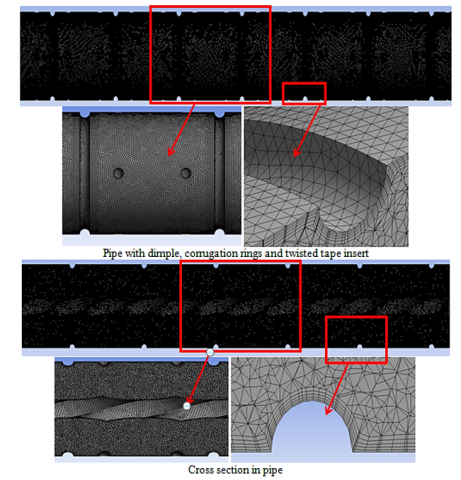Figure 2. Mesh domain in different pipe parts

3.1 Boundary flow conditions

ANSYS FLUENT is utilized to arithmetic computational of a 1920 mm smooth and pipe with different configurations flow model. The mesh domains are generated in the CFD technique environment as well as all information obtained is also investigated by CFD software. Range of Re Number from 1000 to 15000, The Tin is consistent at 313.15 K. The properties of water flow thermophysical are changing with temperature. The pressure fields and acceleration speed are coupled using a simplified algorithm with 2nd order upwind schemes are used. Boundary conditions are inlet velocity with outlet pressure at the outlet condition.

3.2 Mathematical model

A 3D numerical model for smooth and corrugated both pipes has been analyzed. Equations of hydrodynamic flow, thermal flow, and the heat transfer, are calculated in numerical solver, water properties as a working fluid. to investigate the turbulent flow in the pipe. The equations of continuity, momentum, and energy can be expressed are as follows.

Criteria of Governing Equations

The equations of governing to solve the velocity and temperature can be presented as follows.

Mass conservation:

$\frac{\partial\left(\rho u_{i}\right)}{\partial x_{i}}=0$    (1)

Momentum conservation:

$\frac{\partial}{\partial x_{i}}\left[\left(\mu+\mu_{t}\right)\left(\frac{\partial u_{i}}{\partial x_{j}}+\frac{\partial u_{j}}{\partial x_{i}}-\delta_{i j} \frac{2}{3} \frac{\partial u_{k}}{\partial x_{k}}\right)-\rho u_{j} u_{i}+\delta_{i j} p\right]=0$     (2)

Energy conservation:

$\begin{array}{r} \frac{\partial}{\partial x_{j}}\left[\left(k+\frac{\mu_{t} C_{P}}{\sigma_{\tau}}\right) \frac{\partial T}{\partial x_{j}}-\rho u_{J} C_{P} T-\mu_{I} \frac{\partial u_{i}}{\partial x_{j}}\left(\frac{\partial u_{i}}{\partial x_{j}}+\frac{\partial u_{j}}{\partial x_{i}}-\right.\right. \\ \left.\left.\delta_{i j} \frac{2}{3} \frac{\partial u_{k}}{\partial x_{k}}\right)-\rho \varepsilon\right]=0 \end{array}$     (3)

Turbulent kinetic energy (k):

$\frac{\partial}{\partial x_{j}}\left(\rho k u_{j}\right)=\frac{\partial}{\partial x_{j}}\left[\left(\mu+\frac{\mu_{t}}{\sigma_{k}}\right) \frac{\partial k}{\partial x_{j}}\right]+\mu_{t}\left(\frac{\partial u_{i}}{\partial x_{j}}+\frac{\partial u_{j}}{\partial x_{i}}\right) \frac{\partial u_{j}}{\partial x_{i}}-\rho \varepsilon$     (4)

Turbulent kinetic energy dissipation rate (ε):

$\frac{\partial}{\partial x_{j}}\left(\rho \varepsilon u_{j}\right)=\frac{\partial}{\partial x_{j}}\left[\left(\mu+\frac{\mu_{t}}{\sigma_{\varepsilon}}\right) \frac{\partial \varepsilon}{\partial x_{j}}\right]+\rho C_{2} \frac{\varepsilon^{2}}{k+\sqrt{v \varepsilon}}$     (5)

where, ­στ, σ­ε and σk are dissipation and energy rate, turbulent kinetic energy and effective Prandtl numbers respectively, μ­­t represented the eddy flow viscosity can be determined as:

$\mu_{t}=\rho C_{\mu} \frac{k^{2}}{\varepsilon}$      (6)

3.3 Analysis heat transfer equations

The following section includes the dimensionless numbers and equations presented the turbulent heat transfer characteristic and pressure drop in smooth and corrugated pipes heat exchanger.

Reynolds number:

$R_{e}=\frac{\rho D_{h} u}{\mu}$     (7)

where, u and Dh are the mean velocity and hydraulic pipe diameter.

Also, the heat transfer coefficient can be determined as:

$\mathrm{h}=\frac{\mathrm{q}}{\left(T_{w}-T_{b}\right)}$     (8)

The value of the Nusselt number is defined as:

$N_{u}=\frac{\mathrm{h} D_{h}}{\mathrm{k}}$    (9)

where, k represented the fluid thermal conductivity. Moreover, the factor of Darcy friction is expressed as:

$f=\frac{2 \Delta_{P}}{\rho u^{2}} \frac{D_{h}}{L}$     (10)

where, Δp and L are the pressure drop and pipe length.

The overall thermal performance factor PEF is determined as :

$\mathrm{PEF}=\frac{\left(\frac{N_{u}}{N u_{s}}\right)}{\left(f / f_{s}\right)^{1 / 3}}$     (11)

where the subscript s in above equation refers to smooth pipe.

4. Validation

In numerical technique to simulate flow in the pipe with turbulent flows, first should be validated to ensure the current numerical outcomes accuracy. Accordingly, computational calculations result in the pipe are validated and compared with experimental outcomes conducted by Albanesi et al. . Figure 3 represented this comparison (between the experimental and numerical data) under various Re number range. It is perceived that the computational outcomes practically agree well with the rate of heat transfer. As seen that experimental and numerical results agreement can confirm the simulation technique reliability, which adopts in this investigation. The deviation errors are observed less than 6% compared with the Albanesi et al.  findings. So, the present numerical outcomes are very close to the experimental one.

## 3.png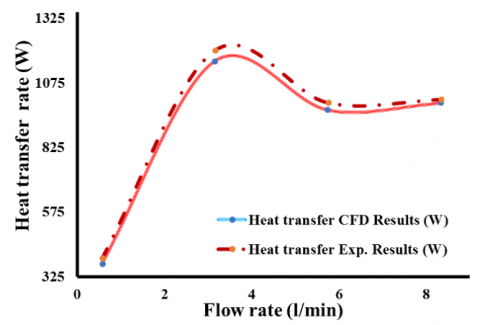Figure 3. Comparison validation results between experimental and numerical data

5. Results and Discussion of Parametrical Investigations

5.1 Flow behaviour features

In this research, different geometrical configurations parameters of investigate the pipe combined with dimple, corrugation ring and twisted insert are analysed numerically. Five twisted insert type configurations are investigated in order to analyse the flow development and thermal performance enhancement. The inner pipe diameter is D = 11.08 mm, corrugated ring diameter is d2 = 1 mm, and the dimple diameter is 0.5 mm in the studied models. The investigated twisted tape insert configurations are the width and thickness of the tape. The following width and thickness of twisted tape values are investigated all conditions to study the influences of these parameters on flow behaviour and thermos-hydraulic performance as well as the enhancement of heat transfer. Water is used as working fluids in the numerical simulation models. The fluid inlet temperature is 313.15 K for the entire of the calculated models with a constant heat flux coefficient of 800 W/m2 in most of the computational simulation. The range of Re number from 1000 to 15000. The dynamic pressure variations under various geometrical configurations are described in Figure 4. As observed in this figure, when the liquid flows passing over the twisted tape, corrugated ring surfaces and dimples, the mixing flow, swirl flow and circulation region are formed near these areas. The maximum value of the dynamic pressure region appeared at the pipe core and hence recognized the circulation zone. The minimum value of pressure appeared close to the pipe wall surfaces as expected due to the low velocity in this zone. Using the different passive techniques such as corrugation, dimple and twisted tape can cause more disturbance in flow stability and hence leads to more fluctuation behaviour in streamlines flow. Moreover, these distortion flow regions between passive devices and flow inside the pipe essentially play a significant role in dynamic pressure variations and improved heat performance.

## 4.pngFigure 4. The effect of varying of twisted tape on dynamic pressure

Figure 5 illustrates the influences of different passive devises configurations on velocity magnitude changes in the pipe. As noted, that the velocity values changing as the geometrical configuration parameters change. In addition, the velocity variations between the twisted tape surfaces and the pipe core has the maximum value because of change in cross section are. Then, the velocity reduces as the water inside the pipe goes near and closed the pipe wall surfaces. These changes in velocity magnitude has a positive impact on heat transfer amount owing to the flow disturbances and velocity gradient. Appling different passive devices in pipes can cause more circulation region and hence the separation of the shear layer leads to cause more vortices. Consequently, that leads to the enhancement of heat transfer.

## 5.pngFigure 5. The influences of different passive devises configurations on velocity magnitude changing

For more analyzing, Figure 6 shows the effect of various passive devices on vorticity magnitude contours changing. It is noticed that the vorticity incenses due to using different twisted tap, dimples and corrugated rings and that leads to cause more rotational flow and secondary flow. The higher vorticity occurs near the pipe wall surface for all models and it is increased as the twisted tape geometrical configurations increase. In the pipe, the swirl flow, spiral flow and secondary flow mostly occur near the corrugation, dimples and twisted tape surfaces and hence they have more influences on the main flow zone. Moreover, the passive device configurations severely influenced the pipe's fluid flow, which the velocity was accelerated. Variation of geometrical parameters have a high impact on the flow behaviour.

## 6.png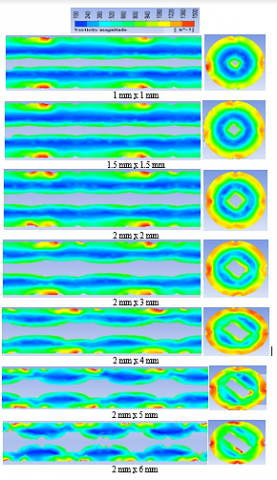Figure 6. The effect of various passive devices on vorticity magnitude changing

Figure 7 represents the turbulent kinetic energy (TKE) distribution contours. The TKE distribution is utilized to show the turbulent fluctuation intensity. TKE variations are strengthened near the outer pipe wall between the twisted tape and pipe wall due to the vortex, swirl and secondary flows. With the twisted tape configurations, change the velocity changes and hence the turbulent fluctuation increases. This is mainly owing to further fluid particles swirling motion, flow mixing in and secondary flow produces an important improvement of heat transfer.

## 7.pngFigure 7. TKE changing by using different passive devices configurations

5.2 Characteristics of thermal flow

Figure 8 depicts the variations in temperature at different twisted tape configurations. It can be noticed that as the twisted tape geometrical parameters change that leads to the temperature variations also change. As seen that the maximum temperature take place between the twisted tape outlet surface and the wall pipe and then it decreases at the outlet pipe wall. This change in twisted geometrical can lead to change the mixing flow in the pipe and hence can improve the heat transfer.

## 8.1.png## 8.2.pngFigure 8. Variations in temperature at various twisted tape configurations

Figure 9 depicts the characteristics of pressure drop in pipes with varying twisted tape configurations. For these cases, pressure drop increases due to the rise of twisted tape dimensions (width and thickness), leading to more mixing of fluid, secondary flow, and swirl flow inside the pipe and hence that can cause a change in fluid viscous sublayer suppression. Based on the aforementioned, due to the high rates of flow recirculation in pipes are responsible for more pressure drop because of these twisted geometries, as compared to smooth pipes. However, the pressure drop showed in this figure, the twisted tape of 2x4 mm has a higher pressure drop.

## 9.png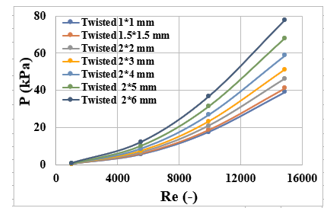Figure 9. Variations in pressure drop with varying Re number ranges

Figure 10 a and b shows variations in both friction factor f and Nu number under varying twisted tape parameters and with various Re number ranges. It is observed that the value of f changes as the tape configurations change. As the tape geometrical parameters increases, the f value also increases due to more variance in velocities flow occur between liquid layers which are adjacent to tape surfaces a pipe wall, as well as pipe core flow layers, become higher. This flow pattern is attributed to more periodic fluid mixing to pipe core. The enhancement extent can change based on the twisted tape variations since the tape geometrical configurations generate more swirls flow which can distort the hydraulic boundary layers and mechanisms of heat performance. Among all investigated models, the higher friction factor according to the smooth pipe reference model is 2x4 mm twisted tape. Correspondingly, compared to the normal pipe, twisted configurations can rise f about 5.4 to 33.5%.

Figure 9 illustrates the effect of twisted tape on Nu with a different range of Re number. It is evidently noted that the value of Nu number increases as the tape geometrical parameters rise. A comparison of results for different twisted dimensions are presented in this figure. It can be noticed that the Nu of these models are changing as the tape dimensions change. This is mostly due to the further mixing and swirling flow motion and the secondary flow can generate significantly higher heat performance. Moreover, it can be observed that chaining in twisted tape inserts configurations have an approximately small effect on Nu value under different geometrical parameters.

For more details, Figure 11 represents the ratio of both f/fo and Nu/Nu under different tape geometrical parameters. As observed in this figure, both ratios have the same trends. These ratios increase as the twisted tape dimensions increase. This happened due to the pipe model with twisted tape has more field of secondary flow comprises much higher flow velocities than that occurs in a smooth pipe. This generates further flow motion in the pipe cross section and hence that leads to an increase the source of heat augmentation based on the tape configurations. Moreover, the common field of secondary flow in a pipe with twisted tape insert is considerably modified to produce swirling flow motion. This common secondary flow involves more oppositely rotating eddies in flow regions. Furthermore, this procedure additional rise in cross sectional the temperature mixing field which can is significant to enhance the overall heat transfer rate.

## 10.pngFigure 10. Variations in f and Nu with varying Re number ranges

## 11.pngFigure 11. Variations in f/fo and Nu/Nuo ratios with different Re number range

Criteria or Thermal Performance

Effective enhanced surface evaluation is the criterium of cost efficient. Hence it is the important criterium for heat improvement. Nevertheless, hydraulic flow criteria and thermal performance essential to be taken into consideration in heat exchangers. The reliability and applicability of these criteria which was employed in different experiments investigations, are the thermal performance efficiencies. The thermal performance coefficient for pipe with varying twisted tape geometrical configurations are shown in Figure 12. A downward tendency can be noted in pipes with twisted tapes owing to the rise in the temperature field gradient in a pipe as well as the working fluid along with pipe, whereas the pipe was exposed to constant heat flux. When liquid passes in the pipe sections, its velocity flow increases owing to the cross-sectional region’s shrinkage. Hence, that leads to an increase in the coefficient of heat transfer near all these regions. After flow liquid passing these areas, more vortex flow is generated near twisted tape surfaces, causing a change in velocity flow and increasing the heat transfer coefficient. Based on this figure, it can clearly be noticed that as the tape configurations increase, that leads to the thermal evaluation factor decreases and the better thermal evaluation factor is at a twisted tape of 1x1 mm at Re number of about 1000. The range value of the thermal evaluation factor is more than 1.67.

## 12.pngFigure 12. Variations in PEF with various Re number ranges

6. Conclusions

Various twisted geometrical configurations are investigated to enhance the heat performance rate. The deviation errors are observed by less than 6% compared with the normal pipe. Pressure drop increases due to twisted tape dimensions, leading to more mixing of fluid, secondary flow, and swirl flow inside the pipe. As the tape geometrical parameters increase, the f value also increases due to more variance in velocities flow between liquid layers, the different flow rate important impacts the flow pattern, the velocity variations between the twisted tape surfaces and the pipe core have the maximum value because of the cross section change. The velocity reduces as the water inside the pipe goes near and closed the pipe wall surfaces. According to the smooth pipe reference model, the higher friction factor is 2x4 mm twisted tape. Compared to the normal pipe, twisted configurations can rise f about 5.4 to 33.5%. The better thermal evaluation factor is at the twisted tape of 1x1 mm at a Re number of about 1000. The range value of the thermal evaluation factor is more than 1.67.

Acknowledgments

In this current analysis, the authors want to acknowledge both the Mustansiriyah University Baghdad – Iraq (www.uomustansiriyah.edu.iq) and Washington State University for their support.

References

 Kareem, Z.S., Jaafar, M.M., Lazim, T.M., Abdullah, S., Abdulwahid, A.F. (2015). Passive heat transfer enhancement review in corrugation. Experimental Thermal and Fluid Science, 68: 22-38. https://doi.org/10.1016/j.expthermflusci.2015.04.012

 Liu, S., Sakr, M. (2013). A comprehensive review on passive heat transfer enhancements in pipe exchangers. Renewable and Sustainable Energy Reviews, 19: 64-81. https://doi.org/10.1016/j.rser.2012.11.021

 Al-Obaidi, A.R. (2020). Investigation of the influence of various numbers of impeller blades on internal flow field analysis and the pressure pulsation of an axial pump based on transient flow behavior. Heat Transfer, 49(4): 2000-2024.‏ https://doi.org/10.1002/htj.21704

 Rainieri, S., Pagliarini, G. (2002). Convective heat transfer to temperature dependent property fluids in the entry region of corrugated tubes. International Journal of Heat and Mass Transfer, 45(22): 4525-4536. https://doi.org/10.1016/S0017-9310(02)00156-4

 Wu, H., Cheng, H., Zhou, Q. (2000). Compound enhanced heat transfer inside tubes by combined use of spirally corrugated tubes and inlet axial vane swirlers. Journal of Enhanced Heat Transfer, 7(4). https://doi.org/10.1615/JEnhHeatTransf.v7.i4.30

 Al-Obaidi, A.R. (2021). Study the influence of concavity shapes on augmentation of heat-transfer performance, pressure field, and fluid pattern in three-dimensional pipe. Heat Transfer. https://doi.org/10.1002/htj.22079

 Al-Obaidi, A.R. (2019). Investigation of fluid field analysis, characteristics of pressure drop and improvement of heat transfer in three-dimensional circular corrugated pipes. Journal of Energy Storage, 26: 101012.‏ https://doi.org/10.1016/j.est.2019.101012

 Al-Obaidi, A.R., Sharif, A. (2020). Investigation of the three-dimensional structure, pressure drop, and heat transfer characteristics of the thermohydraulic flow in a circular pipe with different twisted-tape geometrical configurations. Journal of Thermal Analysis and Calorimetry, 1-26.‏ https://doi.org/10.1007/s10973-019-09244-y

 Al‐Obaidi, A.R. (2020). Analysis of the flow field, thermal performance, and heat transfer augmentation in circular tube using different dimple geometrical configurations with internal twisted‐tape insert. Heat Transfer, 49(8): 4153-4172.‏ https://doi.org/10.1002/htj.21821

 Farshad, S.A., Sheikholeslami, M. (2019). Nanofluid flow inside a solar collector utilizing twisted tape considering exergy and entropy analysis. Renewable Energy, 141: 246-258.‏ https://doi.org/10.1016/j.renene.2019.04.007

 Akhavan-Behabadi, M.A., Kumar, R., Mohammadpour, A., Jamali-Asthiani, M. (2009). Effect of twisted tape insert on heat transfer and pressure drop in horizontal evaporators for the flow of R-134a. International Journal of Refrigeration, 32(5): 922-930. https://doi.org/10.1016/j.ijrefrig.2008.11.004

 Al-Obaidi, A.R. (2021). Investigation of the flow, pressure drop characteristics, and augmentation of heat performance in a 3D flow pipe based on different inserts of twisted tape configurations. Heat Transfer. https://doi.org/10.1002/htj.22115

 Akhavan-Behabadi, M.A., Kumar, R., Mohammadpour, A., Jamali-Asthiani, M. (2009). Effect of twisted tape insert on heat transfer and pressure drop in horizontal evaporators for the flow of R-134a. International Journal of Refrigeration, 32(5): 922-930. https://doi.org/10.1016/j.ijrefrig.2008.11.004

 Alhamid, J., Al-Obaidi, A.R. (2021). Effect of concavity configuration parameters on hydrodynamic and thermal performance in 3D circular pipe using Al2O3 nanofluid based on CFD simulation. In Journal of Physics: Conference Series, 1845(1): 012060. https://doi.org/10.1088/1742-6596/1845/1/012060

 Chen, J., Müller-Steinhagen, H., Duffy, G.G. (2001). Heat transfer enhancement in dimpled tubes. Applied Thermal Engineering, 21(5): 535-547. https://doi.org/10.1016/S1359-4311(00)00067-3

 Kukulka, D.J., Smith, R. (2013). Thermal-hydraulic performance of Vipertex 1EHT enhanced heat transfer tubes. Applied Thermal Engineering, 61(1): 60-66. https://doi.org/10.1016/j.applthermaleng.2012.12.037

 Liao, Q., Xin, M.D. (2000). Augmentation of convective heat transfer inside tubes with three-dimensional internal extended surfaces and twisted-tape inserts. Chemical Engineering Journal, 78(2-3): 95-105. https://doi.org/10.1016/S1385-8947(00)00134-0

 Patil, A.G. (2000). Laminar flow heat transfer and pressure drop characteristics of power-law fluids inside tubes with varying width twisted tape inserts. J. Heat Transfer, 122(1): 143-149. https://doi.org/10.1115/1.521448

 Prasad, P.D., Gupta, A.V.S.S.K.S. (2016). Experimental investigation on enhancement of heat transfer using Al2O3/water nanofluid in a u-tube with twisted tape inserts. International Communications in Heat and Mass Transfer, 75: 154-161. https://doi.org/10.1016/j.icheatmasstransfer.2016.03.019

 Ayub, Z.H., Al-Fahed, S.F. (1993). The effect of gap width between horizontal tube and twisted tape on the pressure drop in turbulent water flow. International Journal of Heat and Fluid Flow, 14(1): 64-67. https://doi.org/10.1016/0142-727X(93)90041-K

 Zimparov, V. (2004). Prediction of friction factors and heat transfer coefficients for turbulent flow in corrugated tubes combined with twisted tape inserts. Part 1: friction factors. International Journal of Heat and Mass Transfer, 47(3): 589-599. https://doi.org/10.1016/j.ijheatmasstransfer.2003.08.004

 Saha, S.K., Dutta, A., Dhal, S.K. (2001). Friction and heat transfer characteristics of laminar swirl flow through a circular tube fitted with regularly spaced twisted-tape elements. International Journal of Heat and Mass Transfer, 44(22): 4211-4223. https://doi.org/10.1016/S0017-9310(01)00077-1

 Thianpong, C., Eiamsa-Ard, P., Wongcharee, K., Eiamsa-Ard, S. (2009). Compound heat transfer enhancement of a dimpled tube with a twisted tape swirl generator. International Communications in Heat and Mass Transfer, 36(7): 698-704. https://doi.org/10.1016/j.icheatmasstransfer.2009.03.026

 Promvonge, P., Eiamsa-ard, S. (2007). Heat transfer behaviors in a tube with combined conical-ring and twisted-tape insert. International Communications in Heat and Mass Transfer, 34(7): 849-859. https://doi.org/10.1016/j.icheatmasstransfer.2007.03.019

 Eiamsa-Ard, S., Thianpong, C., Eiamsa-Ard, P., Promvonge, P. (2009). Convective heat transfer in a circular tube with short-length twisted tape insert. International Communications in Heat and mass Transfer, 36(4): 365-371. https://doi.org/10.1016/j.icheatmasstransfer.2009.01.006

 Zhang, X., Liu, Z., Liu, W. (2013). Numerical studies on heat transfer and friction factor characteristics of a tube fitted with helical screw-tape without core-rod inserts. International Journal of Heat and Mass Transfer, 60: 490-498. https://doi.org/10.1016/j.ijheatmasstransfer.2013.01.041

 Ray, S., Date, A.W. (2003). Friction and heat transfer characteristics of flow through square duct with twisted tape insert. International Journal of Heat and Mass Transfer, 46(5): 889-902. https://doi.org/10.1016/S0017-9310(02)00355-1

 Al-Obaidi, A.R., Alhamid, J. (2021). Numerical investigation of fluid flow, characteristics of thermal performance and enhancement of heat transfer of corrugated pipes with various configurations. In Journal of Physics: Conference Series, 1733(1): 012004. https://doi.org/10.1088/1742-6596/1733/1/012004

 Bellos, E., Tzivanidis, C. (2018). Enhancing the performance of evacuated and non-evacuated parabolic trough collectors using twisted tape inserts, perforated plate inserts and internally finned absorber. Energies, 11(5): 1129. https://doi.org/ 10.1016/j.rser.2018.03.091

 Salman, S.D., Kadhum, A.A.H., Takriff, M.S., Mohamad, A.B. (2014). CFD simulation of heat transfer augmentation in a circular tube fitted with alternative axis twisted tape in laminar flow under a constant heat flux. Heat Transfer—Asian Research, 43(4): 384-396. https://doi.org/10.1002/htj.21089

 Albanesi, A.W., Daish, K.D., Dally, B., Chin, R.C. (2018). Investigation of heat transfer enhancement in dimpled pipe flows. 21st Australasian Fluid Mechanics Conference Adelaide, Australia 2018.